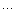# BaSaRa 目前关心的是UI技术

## [引用] 对闭包的理解

Public变量：
function Test()
{

this.x = 1;
}

Test.prototype.x = 1;

Test.prototype.Plus = function () {}

Private变量：
function Test()
{

var self = this;

var x = 1;

function Plus1() {}

var pPlus = function Plus2() {}
}

Privileged变量：
function Test()
{

this.pPlus = function () {}
}

<html>

<body>

<input type="button" id="test" value="test" />

<script>
function Test (x, y)
{

var x = x;

var y = y;
}
= function ()
{
this.x + this.y);
}

var t = new Test(12);
document.getElementById('test').onclick

</script>

</body>
</html>

<html>

<body>

<input type="button" id="test" value="test" NAME="test"/>

<script>
function Test (x, y)
{

var x = x;

var y = y;

this.add = function ()    // 特权变量，可以访问私有变量，又对外公开
{
+ y);
}
}

var t = new Test(12);
document.getElementById('test').onclick

</script>

</body>
</html>

<html>

<body>

<input type="button" id="test" value="test" NAME="test"/>

<script>
function Test ()
{

var z = 1;

{
++);
}
}

var t = new Test();
document.getElementById('test').onclick

</script>

</body>
</html>

<html>

<body>

<input type="button" id="test" value="test" NAME="test"/>

<script>
function Test ()
{

var z = 1;

var self = this;

var pBtn = null;

this.selfSubtract = function ()
{
z
--;z--;
pBtn.onclick
= self.selfPlus;
}

this.selfPlus = function ()
{
z
++;
pBtn.onclick
= self.selfSubtract;
}

this.getFunction = function (btn)
{
pBtn
= btn;

return self.selfSubtract;
}
}

var t = new Test();
var btn = document.getElementById('test');
btn.onclick
= t.getFunction(btn);

</script>

</body>
</html>

posted on 2006-06-13 11:13 BaSaRa 阅读(224) 评论(0)  编辑  收藏 所属分类: Javascript只有注册用户登录后才能发表评论。 网站导航: 相关文章:

• 随笔 - 14
• 文章 - 0
• 评论 - 3

•